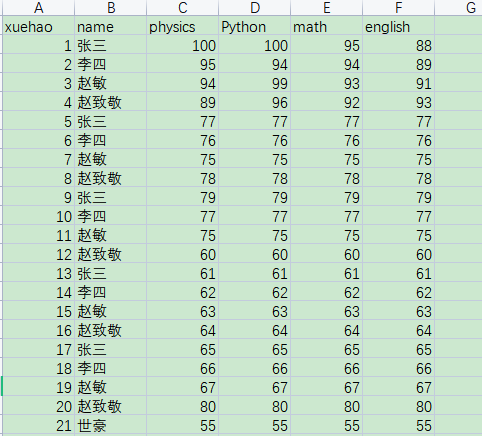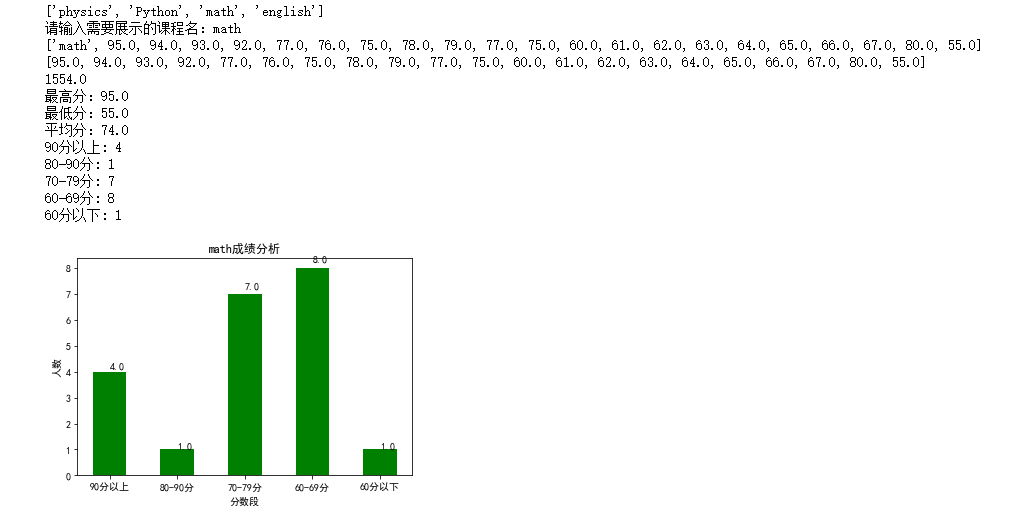• 学生成绩数据分析 FCC-BUAP数据集的基础数据分析。 优胜劣汰的案例研究，综合实绩研究，学生表现分析.pdf Nombre del数据集：学生成绩数据集 说明：此数据用于评估两所葡萄牙学校的中等学生的学习成绩。 数据...R
• 中学学生成绩数据分析系统，输入成绩，系统自动按要求输出结果
• 本程序从Excel文件读取学生成绩，统计各个分数段（90分以上，8089分，7079分，60~69分，60分以下）学生人数，并用柱状图（如图9-1）展示学生成绩分布，同时计算出最高分、最低分、平均成绩、各分数段人数等分析指标...


文章目录
题目要求1. 建一个数据测试表2. 编写程序3. 效果展示

题目要求
学生成绩存储在Excel文件（如表9-1）中，本程序从Excel文件读取学生成绩，统计各个分数段（90分以上，8089分，7079分，60~69分，60分以下）学生人数，并用柱状图（如图9-1）展示学生成绩分布，同时计算出最高分、最低分、平均成绩、各分数段人数等分析指标。
1. 建一个数据测试表
随便建，最好各个分数区间都有，展示出来更直观，示例建表如下：2. 编写程序
程序可分为两部分编写，一部分用于读取表格数据，拿到分数数据再求其他相关量就好做了，第二部分就是拿到第一部分的成绩列表，再进行具体的数据分析需求。 示例代码：
#!/usr/bin/env python3
# -*- coding: utf-8 -*-
import xlrd
import matplotlib.pyplot as plt

wb = xlrd.open_workbook('mark.xlsx')
sheet = wb.sheet_names()
sh = wb.sheet_by_name('Sheet1')
course_list = sh.row_values(0)
print(course_list[2:])  # ['physics', 'Python', 'math', 'english']
course = input('请输入需要展示的课程名：')
m = course_list.index(course)
col_list = sh.col_values(m)
print(col_list)  # ['math', 95.0, 94.0, 93.0, 92.0]
score_list = col_list[1:]
print(score_list)
print(sum(score_list))
print('最高分：%s ' % max(score_list))
print('最低分：%s' % min(score_list))
print('平均分：%s' % (int(sum(score_list)) / len(score_list)))

y = [0, 0, 0, 0, 0]
for score in score_list:
if score >= 90:
y += 1
elif score >= 80:
y += 1
elif score >= 70:
y += 1
elif score >= 60:
y += 1
else:
y += 1
print('90分以上:',y)
print('80-90分:',y)
print('70-79分:',y)
print('60-69分:',y)
print('60分以下:',y)

xl = ['90分以上', '80-90分', '70-79分', '60-69分', '60分以下']

x = [1, 2, 3, 4, 5]
plt.xlabel('分数段')
plt.ylabel('人数')
plt.rcParams['font.sans-serif'] = ['SimHei']
plt.xticks(x, xl)
recs = plt.bar(x=x, height=y, color='green', width=0.5)
plt.title(course + '成绩分析')
for rec in recs:
height = rec.get_height()
plt.text(rec.get_x() + rec.get_width() / 2.0, 1.03 * height, '%s' % float(height))

plt.show()


3. 效果展示展开全文• 基于关联分析的高校护理专业学生成绩数据挖掘与分析研究.pdf
• 目标：分析学生成绩的影响因素 1.导入原始数据，以及需要用到的库 import pandas as pd import numpy as np df = pd.read_csv('StudentsPerformance.csv') (数据来源于kaggle) 2.查看文件 从上面的信息可以看出这...
目标：分析学生成绩的影响因素
1.导入原始数据，以及需要用到的库
import pandas as pd
import numpy as np

(数据来源于kaggle)
2.查看文件从上面的信息可以看出这一千个学生的数据中是没有空值，而且可以看出各列数据的类型。 还可以通过unique来查看某列数据都有哪些值，是否有无效数据。
df['parental level of education'].unique()
df['test preparation course'].unique()再来查看成绩是否为有效值（使用loc）
df.loc[(df['math score']<0) | (df['math score']>100) | (df['reading score']<0) | (df['reading score']>100) | (df['writing score']<0) | (df['writing score']>100)]可以看出成绩中没有无效值
3.数据处理 根据目标，删除一些不需要的列（使用drop）
df.drop(['gender','race/ethnicity'],axis=1)
#参数axis默认值为0，指行，要删除列的话将axis设为1
df_1 = df.drop(['gender','race/ethnicity'],axis=1)求学生成绩平均值
df_1['average']= round((df_1['math score']+df_1['reading score']+df_1['writing score'])/3)
#round()用来对数据四舍五入4.分析学生成绩影响因素
df_1.groupby('parental level of education')['average'].agg([np.mean]).plot.bar()
df_1.groupby('lunch')['average'].agg([np.mean]).plot.bar()
df_1.groupby('test preparation course')['average'].agg([np.mean]).plot.bar()从上面三张图可以初步得出结论： 1.父母学历越高，学生成绩越好 2.午餐吃的好的同学成绩较高 3.考试准备充分的同学成绩较高
以下，用相关系数分析父母学历对哪科成绩影响最大
先按照父母学历高低将表中数据替换为数值
df_1.replace({'some high school':1, 'high school':2,'some college':3,"associate's degree":4,"bachelor's degree":5,"master's degree":6},inplace = True)
#inplace = True是为了改变文档的源数据用corr求出相关系数（相关系数接近0，说明相关性小，越接近1，正相关性越强，越接近-1,负相关性越强）根据父母学历与学生数学、阅读、写作成绩的相关系数，可以看出，父母学历与三者都成正相关，其中与写作成绩相关性最强，而且各科之间相关性都很强，说明某一科成绩好的同学其他科成绩很可能也好。
5.结论 通过以上分析，可以初步得出以下的结论： 1.父母学历越高，学生成绩越好（对写作成绩的影响最大） 2.午餐吃的好的同学成绩较高 3.考试准备充分的同学成绩较高 4.某科成绩好的学生其他科成绩也较好（其中写作与阅读成绩相关性最强）
展开全文• 利用Python分析学生成绩一、题目描述二、解题步骤运行环境题目分析三、完整代码四、运行结果五、参考文献 一、题目描述 个人构造一个30名学生的班级，每名学生有三门课程，学生的学号和各门成绩形如： 学号 高数 ...


利用Python分析学生成绩
一、题目描述二、解题步骤运行环境题目分析
三、完整代码四、运行结果五、参考文献附录附录A更新记录
附录B 相关练习题目练习一：读取Excel文件数据表分别存入DataFrame对象Score和Duty练习二：自定义数据，完成直方图，折线图，散点图，饼图等

一、题目描述
个人构造一个30名学生的班级，每名学生有三门课程，学生的学号和各门成绩形如：
学号 高数 英语 Python 1001 85 90 96 1002 96 92 95 1003 78 87 83 为了输入数据方便，请保存在如student_score.csv文件中。 （1）请计算三门课程的总分，此班级每门课程的平均分和最高分及最低分，并绘制相应的图形来统计三门课程的成绩分布。 （2）各图形自拟。 （3）坐标轴标签，图例等属性设置完整。 （4）使用中文标题及标签。
二、解题步骤
运行环境
Anconda3.X Spyder（Python 3.7）
题目分析
步骤一：创建csv文件以保存实验所需数据。 本文使用使用Excel创建CSV文件。操作步骤如下： 1）新建一个Excel表，（使用WPS和Microsoft都是一样的） 2）打开Excel进行编辑。 3）生成.csv文件。 方式一：WPS表格-另存为-其它格式-选择文件类型-命名文件名，最后保存。 方式二：保存文档后直接修改“新建 Microsoft Excel 工作表.xlsx”为“使用Excel创建CSV文件.csv”。 4）点击保存后有相应警告提醒，则选“确定”或“是”即可
本文使用到的资源（百度网盘） 链接：https://pan.baidu.com/s/1gKDtK2yNfPgj_2OIzWI0TA 提取码：8vnx
步骤二：导入所需要的库
import pandas as pd	#进行文件读取
import matplotlib.pyplot as plt #绘图

步骤三：读取csv文件 在Spyder中读取student_score.csv为DataFrame字符流，并且赋值给df，需要设置编码格式为GBK格式。本文将数据文件放置在同一目录下。作者在使用UFT-8编码会无法正确读出数据。
score = pd.read_csv('./student_score.csv',encoding = 'gbk')#这里使用的是相对路径
#获取文件绝对路径
#import os
#file_path = os.path.abspath('students_score.csv')
#print(file_path)

步骤四：提取所需数据 此步骤中涉及到DataFrame的一些操作。本文在提取相关数据时使用访问属性的方式访问DataFrame单列数据。在附录B中有使用字典访问内部数据的方式访问DataFrame单列数据。
score = pd.read_csv('./student_score.csv',encoding = 'gbk')
#最高分
Math_Max = score.高数.max()
English_Max = score.英语.max()
Python_Max = score.Python.max()
#最低分
Math_Min = score.高数.min()
English_Min = score.英语.min()
Python_Min = score.Python.min()
#成绩方差
Math_Var = score.高数.var()
English_Var = score.英语.var()
Python_Var = score.Python.var()
#成绩均值
Math_Avg = score.高数.mean()
English_Avg = score.英语.mean()
Python_Avg = score.Python.mean()
#学生总成绩
Total_Score = score.高数 + score.英语 + score.Python

步骤五：设置字体 由于默认的pyplot字体并不支持中文字符的显示，因此需要通过设置font.sans-serif参数来改变绘图时的字体，使得可以正常显示中文。同时，由于更改字体后会导致坐标轴中的字体无法显示，因此要同时更改axes.unicode_minus参数
plt.rcParams['font.sans-serif']=['SimHei']
plt.rcParams['axes.unicode_minus']=False

步骤六：数据可视化 本文只列出了所有学生总成绩的直方图，折线图，散点图和每门课程的箱线图，其他部分的图形数据较少只给出直方图。其他的绘图在附录B中有相关代码参考。
plt.title('学生总成绩分布直方图')
plt.xlabel('学号')
plt.ylabel('总分')
plt.bar(score.学号,Total_Score)
plt.show()

plt.title('学生总成绩分布折线图')
plt.xlabel('学号')
plt.ylabel('总分')
plt.plot(score.学号,Total_Score)
plt.show()

plt.title('学生总成绩分布散点图')
plt.xlabel('学号')
plt.ylabel('总分')
plt.scatter(score.学号,Total_Score)
plt.show()

plt.title('每门课程箱线图')
plt.xlabel('课程名')
plt.ylabel('分数')
label = ['高数','英语','Python']
s = (score.高数,score.英语,score.Python)
plt.boxplot(s,labels = label)
plt.show()

plt.title('每门课程平均分直方图')
plt.xlabel('课程名')
plt.ylabel('平均分')
plt.bar('Python',Python_Avg)
plt.bar('高数',Math_Avg)
plt.bar('英语',English_Avg)
plt.show()

plt.title('每门课程最高分直方图')
plt.xlabel('课程名')
plt.ylabel('最高分')
plt.bar('Python',Python_Max)
plt.bar('高数',Math_Max)
plt.bar('英语',English_Max)
plt.show()

plt.title('每门课程最低分直方图')
plt.xlabel('课程名')
plt.ylabel('最低分')
plt.bar('Python',Python_Min)
plt.bar('高数',Math_Min)
plt.bar('英语',English_Min)
plt.show()

也可以通过创建子图的方式显示，此时需要创建画布。
p1=plt.figure()#创建画布
plt.title('学生总成绩分布直方图')
plt.xlabel('学号')
plt.ylabel('总分')
plt.bar(score.学号,Total_Score)

plt.title('学生总成绩分布折线图')
plt.xlabel('学号')
plt.ylabel('总分')
plt.plot(score.学号,Total_Score)

plt.title('学生总成绩分布散点图')
plt.xlabel('学号')
plt.ylabel('总分')
plt.scatter(score.学号,Total_Score)

plt.title('每门课程箱线图')
plt.xlabel('课程名')
plt.ylabel('分数')
label = ['高数','英语','Python']
s = (score.高数,score.英语,score.Python)
plt.boxplot(s,labels = label)
plt.show()

三、完整代码
import pandas as pd
import matplotlib.pyplot as plt

#读取文件

#最高分
Math_Max = score.高数.max()
English_Max = score.英语.max()
Python_Max = score.Python.max()
#最低分
Math_Min = score.高数.min()
English_Min = score.英语.min()
Python_Min = score.Python.min()
#成绩方差
Math_Var = score.高数.var()
English_Var = score.英语.var()
Python_Var = score.Python.var()
#成绩均值
Math_Avg = score.高数.mean()
English_Avg = score.英语.mean()
Python_Avg = score.Python.mean()
#学生总成绩
Total_Score = score.高数 + score.英语 + score.Python

plt.rcParams['font.sans-serif']=['SimHei']
plt.rcParams['axes.unicode_minus']=False

plt.title('学生总成绩分布直方图')
plt.xlabel('学号')
plt.ylabel('总分')
plt.bar(score.学号,Total_Score)
plt.show()

plt.title('学生总成绩分布折线图')
plt.xlabel('学号')
plt.ylabel('总分')
plt.plot(score.学号,Total_Score)
plt.show()

plt.title('学生总成绩分布散点图')
plt.xlabel('学号')
plt.ylabel('总分')
plt.scatter(score.学号,Total_Score)
plt.show()

plt.title('每门课程箱线图')
plt.xlabel('课程名')
plt.ylabel('分数')
label = ['高数','英语','Python']
s = (score.高数,score.英语,score.Python)
plt.boxplot(s,labels = label)
plt.show()

plt.title('每门课程平均分直方图')
plt.xlabel('课程名')
plt.ylabel('平均分')
plt.bar('Python',Python_Avg)
plt.bar('高数',Math_Avg)
plt.bar('英语',English_Avg)
plt.show()

plt.title('每门课程最高分直方图')
plt.xlabel('课程名')
plt.ylabel('最高分')
plt.bar('Python',Python_Max)
plt.bar('高数',Math_Max)
plt.bar('英语',English_Max)
plt.show()

plt.title('每门课程最低分直方图')
plt.xlabel('课程名')
plt.ylabel('最低分')
plt.bar('Python',Python_Min)
plt.bar('高数',Math_Min)
plt.bar('英语',English_Min)
plt.show()

四、运行结果通过创建子图的方式显示：五、参考文献
黄红梅，张良均.Python数据分析与应用[M].北京:人民邮电出版社,2018(4):52-89 python 计算均值、方差、标准差 Numpy，Pandas
附录
附录A
更新记录
2021-05-04
新增：自定义数据，完成直方图，折线图，散点图，饼图等。
使用字典访问内部数据的方式访问DataFrame单列数据。
读取Excel文件数据表。请参考附录B 相关练习题目
2021-05-24
新增：附录B运行截图。
更改：文中部分描述错误。
2021-06-18
新增：文中正文与附录中的测试代码文件，正文文件为网盘连接。

附录B 相关练习题目
练习一：读取Excel文件数据表分别存入DataFrame对象Score和Duty
题目描述 1.练习pandas统计分析基础，数据读取、分析、输出。 2.自建EXCEL文件，分为2个工作区，分别存放学生信息表（不少于30人）和班级职务表（不少于4种职务）。
如信息表： 学号 姓名 性别 数学 英语 Python 通信技术 1001 张三 男 95 86 87 90 1002 李四 女 98 84 88 89 如班级职务表： 学号 职务 1001 班长 1002 学习委员 读取Excel文件数据表分别存入DataFrame对象Score和Duty。要求如下：
1）Score对象新增一列“总分”为前四列成绩之和。 2）Score对象依据“总分”列的值从高到低进行排序。 3）Score对象根据性别列进行分组，输出男女生各自的平均分。 4）输出男女生的最高分。 5）Score对象新增一列“等级”，总分大于360的等级为A，总分小于270的等级为C，介于270到360之间为B。 6）以“学号”列为关联关键，将Score对象和Duty对象合并，保留所有Score对象的数据行，合并声称新的DataFrame对象Students。 7）把Students对象数据存入新的Excel文件students.xlsx中。
本文用到的数据class.xlsx
参考代码 请注意在#Anaconda 3.7版本中 ‘sheetname’ 命令，已更新为 ‘sheet_name’ 如果有报错：TypeError: read_excel() got an unexpected keyword argument 'sheetname'请尝试将’sheetname‘改为’sheet_name’
import pandas as pd

#TypeError: read_excel() got an unexpected keyword argument sheetname
#Anaconda 3.7版本中 'sheetname' 命令，已更新为 'sheet_name'

Score['总分'] = Score['数学'] + Score['英语'] + Score['Python'] + Score['通信技术']
Score = Score.sort_values(by='总分',ascending=False)

newgroup = Score[['性别','数学','英语','Python','通信技术','总分']].groupby( by  ='性别')
print(newgroup.mean())
print(newgroup.max())

Score['等级'] = Score['总分'].apply(lambda x: 'A' if x>=360 else('C' if x<270  else 'B'))

#print(Score)
#print(Duty)

Students = pd.merge(left=Score , right=Duty , how="outer",on='学号')
#Students = pd.concat([Score,Duty],axis=1,join='inner')
print(Students)

Students.to_excel('./students.xlsx')

运行结果
           数学         英语     Python       通信技术          总分

性别

女   81.823529  88.588235  89.176471  80.941176  340.529412

男   87.722222  88.055556  89.944444  78.833333  344.555556

数学  英语  Python  通信技术   总分

性别

女   99  97      99    91  362

男   97  98      99    94  362

学号 性别  数学  英语  Python  通信技术   总分 等级    职务

0   1035  男  89  95      84    94  362  A  体育委员

1   1030  女  84  90      99    89  362  A  纪律委员

2   1010  男  94  89      99    79  361  A     无

3   1034  男  88  94      83    93  358  B     无

4   1029  女  83  89      98    88  358  B  心理委员

5   1009  男  93  88      98    78  357  B     无

6   1028  女  82  88      97    87  354  B  心理委员

7   1033  男  87  93      82    92  354  B     无

8   1008  男  92  87      97    77  353  B  学习委员

9   1015  女  99  94      84    74  351  B     无

10  1027  男  81  87      96    86  350  B     无

11  1032  女  86  92      81    91  350  B     无

12  1007  男  91  86      96    76  349  B  学习委员

13  1014  女  98  93      83    73  347  B     无

14  1026  女  80  86      95    85  346  B     无

15  1006  男  90  85      95    75  345  B     无

16  1013  男  97  92      82    72  343  B     无

17  1025  女  79  85      94    84  342  B     无

18  1005  男  89  84      94    74  341  B     无

19  1012  男  96  91      81    71  339  B     无

20  1024  女  78  84      93    83  338  B     无

21  1019  男  73  98      88    78  337  B  宣传委员

22  1004  男  88  83      93    73  337  B     无

23  1031  女  85  91      70    90  336  B  纪律委员

24  1011  男  95  90      80    70  335  B     无

25  1023  女  77  83      92    82  334  B     无

26  1018  女  72  97      87    77  333  B     无

27  1003  男  87  82      92    72  333  B    班长

28  1022  女  76  82      91    81  330  B     无

29  1002  女  86  81      91    71  329  B     无

30  1017  女  71  96      86    76  329  B     无

31  1021  男  75  81      90    80  326  B  心理委员

32  1016  女  70  95      85    75  325  B     无

33  1001  女  85  80      90    70  325  B     无

34  1020  男  74  80      89    79  322  B  宣传委员

练习二：自定义数据，完成直方图，折线图，散点图，饼图等
参考代码
import pandas as pd
import matplotlib.pyplot as plt

stu_Score = stu['数学'] + stu['英语'] + stu['Python'] + stu['通信技术']
stu['总分'] = stu_Score
print(stu)

plt.rcParams['font.sans-serif']=['SimHei']
plt.rcParams['axes.unicode_minus']=False

plt.title('学生总成绩分布直方图')
plt.xlabel('学号')
plt.ylabel('总分')
plt.bar(stu['学号'],stu['总分'])
plt.show()

plt.title('学生成绩分布折线图')
plt.xlabel('学号')
plt.ylabel('分数')
plt.plot(stu['学号'],stu['总分'],'r-',stu['学号'],stu['数学'],'b-',stu['学号'],stu['英语'],'y--',
stu['学号'],stu['Python'],'g',stu['学号'],stu['通信技术'],'m-')
plt.legend(['总分','数学','英语','Python','通信技术'])
plt.show()

plt.title('学生成绩分布散点图')
plt.xlabel('学号')
plt.ylabel('分数')
plt.scatter(stu['学号'],stu['总分'])
plt.scatter(stu['学号'],stu['数学'])
plt.scatter(stu['学号'],stu['英语'])
plt.scatter(stu['学号'],stu['Python'])
plt.scatter(stu['学号'],stu['通信技术'])
plt.legend(['总分','数学','英语','Python','通信技术'])
plt.show()

plt.title('学生成绩饼图')
label = ['数学','英语','Python','通信技术']
X = [stu['数学'].sum(),stu['英语'].sum(),stu['Python'].sum(),stu['通信技术'].sum()]
plt.pie(X,labels=label,autopct='%1.2f%%')
plt.show()

运行结果展开全文python csv
• 此数据集含有学生3门课程成绩数据学生个人信息、社交信息等信息。共计1000行，每行8种数据。在此分享出来供大家学习使用。
• 基于ID3算法的大学生成绩数据挖掘与体能分析系统设计.pdf
• 采用数据挖掘技术中ID3决策树算法分析学生成绩.pdf 采用数据挖掘技术中ID3决策树算法分析学生成绩.pdf
•  针对课程设置与课程成绩之间的联系，通过比较分析可以发现学生成绩背后所...通过对学生成绩数据的挖掘，可以发现隐藏其中的关联规则，得出有实际价值的规则及结论，从而为教学管理人员优化课程设置提供决策支持。
• 是本科毕业设计，用的是C4.5数据挖掘算法，分析前导课程与后续课程的关系和平时成绩对期末成绩的关系。...